### Solutions manual for NTB - 3.3. Basic integer arithmetic

Let's review some notations and facts. For an integer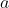, we define its bit length, or simply, its length, which we denote by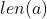, to be the number of bits in the binary representation of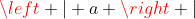; more precisely,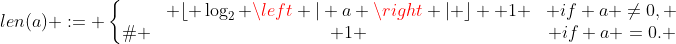If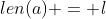, we say thatis an-bit integer. Notice that ifis a positive,-bit integer, then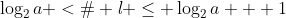, or equivalently,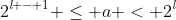.

Let a and b be arbitrary integers. Then we have:

(i) We can compute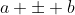in time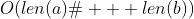.

(ii) We can compute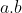in time.

Let a and b be arbitrary integers. Then we have:

(i) We can computein time.

(ii) We can computein time.

(iii) If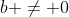, we can compute the quotient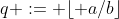and the remainderin time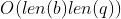.

Exercise 3.24. Let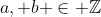with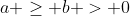, and let. Show that:

Proof

If you look at the
list of errata, you'll see that I found a stronger version of this exercise. My proof is as following.

We see that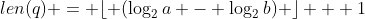. Hence what we need to prove is: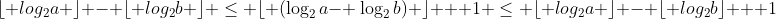which is the same as proving: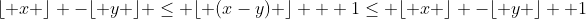for some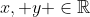which in turn can be proved by using this fact: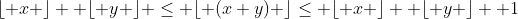for all.

(q.e.d.)

Exercise 3.25. Let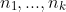be postivie integers. Show that: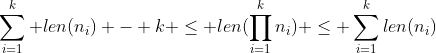Proof

As in Exercise 3.24, I think I found a slightly better version of this exercise as follows: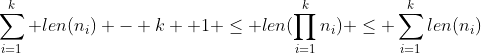We can prove it using the same technique as the proof of Exercise 3.24. We see that: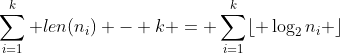,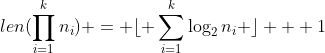,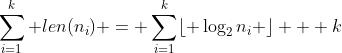Since we havefor all, we see that: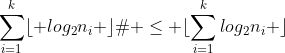and,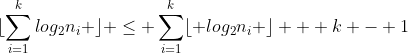.

(q.e.d.)

Exercise 3.26. Show that given integers, with each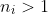, we can compute the product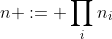in time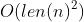.

Proof

(q.e.d.)

Exercise 3.27. Show that given integers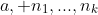, with each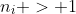, where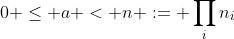, we can compute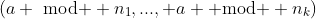in time.

Proof

(q.e.d.)

Exercise 3.28. Show that given integers, with each, we can compute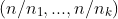, where, in time.

Proof

(q.e.d.)

Exercise 3.29. This exercise develops an algorithm to compute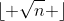for a given positive integer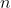. Consider the following algorithm: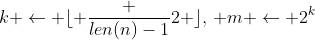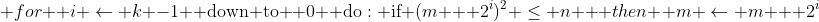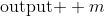(a) Show that this algorithm correctly computes.

(b) In a straightforward implementation of this algorithm, each loop iteration takes time, yielding a total running time of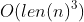. Give a more careful implementation, so that each loop iteration takes time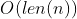, yielding a total running time is.

Proof

(q.e.d.)

Exercise 3.30. Modify the algorithm in the previous exercise so that given positive integersand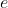, with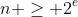, it computes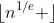in time.

Proof

(q.e.d.)

Exercise 3.31. An integer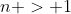is called a perfect power if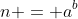for some integers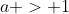and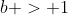. Using the algorithm from the previous exercis, design an efficient algorithm that determines if a givenis perfect power, and if it is, also computesand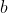such that, where,, andis as small as possible. Your algorithm should run in time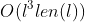where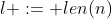.

Proof

(q.e.d.)

Exercise 3.32. Show how to convert (in both directions) in timebetween the base-10 representation and our implementation's internal representation of an integer.

Proof

(q.e.d.)